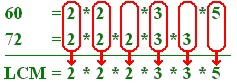# Problems on LCM

## Problems on LCM Shortcut Tricks

Common Multiple: Defined as number which is exactly divisible by each of the numbers under consideration. Example 60 is a common multiple of 2,3,4,5,6,10,15,20 and 30

Lowest Common Multiple(LCM): LCM of two or more numbers is the least or smallest number which is exactly divisible by each of them.

## Solution

Multiples of 12 are 12, 24 ,36, 48, 60 Multiples of 16 are 16, 32, 48, 64 So the least common multiple of both is 48 that is required LCM

### Method of Prime Factors

• Step-1 Resolve each of the given numbers into prime factors
• Step-2 Find the product of the highest powers of all the factors that occour in the resolution of the given number. This product will be required LCM

## Solution

```8= 2*2*2= 2^3

12= 2*2*3= 2^2*3

15=3*5

21=3*7

So Product of prime numbers with highest powers
(2^3)*3*5*7=840 required LCM
```

### Short Cut Method

Write down the given numbers in a line separating them by commas. Divide by any one of prime numbers 2, 3,5,7 which al least exactly divide at least any two of the given numbers . Repeat down the process until you get a line of prime numbers. Now the product of all divisors and the numbers in the last line will be the required LCM.

## Solution

```The multiples of 12 are : 12, 24, 36, 48, 60, 72, 84, ....
The multiples of 15 are : 15, 30, 45, 60, 75, 90, ....
60 is a common multiple (a multiple of both 12 and 15), and there are no lower common multiples.
Therefore, the lowest common multiple of 12 and 15 is 60.
```

## Solution

```We want to find the LCM of the numbers 60 and 72.
Start by writing each number as a product of its prime factors.
60 = 2 * 2 * 3 * 5
72 = 2 * 2 * 2 * 3 * 3
```

To make the next stage easier, we need to write these so that each new prime factor begins in the same place:

## Solution

```60	= 2	* 2		* 3		* 5
72	= 2	* 2	* 2	* 3	* 3
```

All the "2"s are now above each other, as are the "3"s etc. This allows us to match up the prime factors.
The highest common factor is found by multiplying all the factors which appear in both lists:So the LCM of 60 and 72 is 2 × 2 × 2 × 3 × 3 × 5 which is 360.